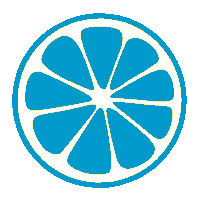#### 若有定义：int a; float b; double c; ，程序运行时输入：a=1,b=2,c=3 <回车>，能把值1输入给变量a、值2输入给变量b、值3输入给变量c的输入语句是

• A.scanf("a=%d,b=%f,c=%lf", &a,&b,&c);
• B.scanf("%d%f%lf", &a,&b,&c);
• C.scanf("a=%d,b=%lf,c=%lf", &a,&b,&c);
• D.scanf("a=%d,b=%f,c=%f", &a,&b,&c);2022年计算机等级考试题库